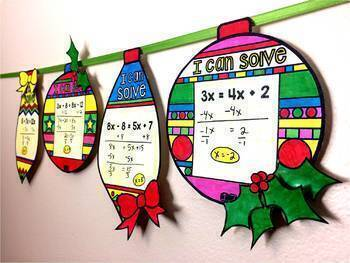# Christmas Algebra Ornaments Bundle Solving Equations & Graphing Linear EquationsGrade Levels
8th - 9th
Subjects
Standards
Resource Type
Formats Included
• Zip
Pages
40 pages
\$6.00
Bundle
List Price:
\$8.00
You Save:
\$2.00
\$6.00
Bundle
List Price:
\$8.00
You Save:
\$2.00
Share this resource

#### Also included in

1. This bundle currently includes 38 algebra pennants and will be updated with additional sets when they are created. You will be notified when a new set is added and will be able to re-download to get the new sets free of charge. Math pennants are a fun way to show off student work, create colorful bu
\$70.00
\$123.50
Save \$53.50

### Description

This bundle includes 60 Christmas math pennants for solving 2-step equations, solving multi-step equations and graphing linear equations. Each pennant is in the shape of an ornament making this a fun activity to complete around the holidays. There are optional student answer sheets, answer keys and blank ornaments included allowing you to write in your own problems. You can read more about each activity below:

Solving Equations Ornaments

Students solve 2-step and multi-step equations in this math pennant activity that doubles as Christmas classroom décor. Equations have variables on the right, left or both sides making the activity easy to differentiate for each student. There are also 4 blank pennants for you to write your own equations.

Included are 30 pennants, an optional student answer sheet and answer key. There are 20 2-step equations of varying difficulty. (Examples are 2x - 4 = 2 and 4 + 3x = 5x.) and 10 multi-step equations with variables on both sides. Includes 4 pennants without equations so that you can write in your own.

Graphing Linear Equations Ornaments

Students graph linear equations and write their equations in this activity that doubles as classroom décor. Question types include:

1: Graphing from slope-intercept form

2: Graphing from point-slope form

3: Graphing & writing the slope-intercept equation given slope and a point

4: Graphing & writing the point-slope equation given slope and a point

5: Graphing & writing the slope-intercept equation given 2 points

6: Graphing & giving a point-slope equation given 2 points

Also includes 4 pennants without equations so that you can write in your own.

Once a pennant is complete, it can be hung along a string in your classroom to show the world that, "Hey, we know how to graph and solve!" Students can also choose to decorate their ornaments with bows and holly that can be cut out from each page. These make for a super festive classroom display.

You may like:

Graphing Linear Equations Digital Math Escape Room

Algebra Word Wall - print and digital

Total Pages
40 pages
Answer Key
Included
Teaching Duration
N/A
Report this Resource to TpT
Reported resources will be reviewed by our team. Report this resource to let us know if this resource violates TpT’s content guidelines.

### Standards

to see state-specific standards (only available in the US).
Understand that the graph of an equation in two variables is the set of all its solutions plotted in the coordinate plane, often forming a curve (which could be a line).
Solve linear equations and inequalities in one variable, including equations with coefficients represented by letters.
Construct a function to model a linear relationship between two quantities. Determine the rate of change and initial value of the function from a description of a relationship or from two (𝘹, 𝘺) values, including reading these from a table or from a graph. Interpret the rate of change and initial value of a linear function in terms of the situation it models, and in terms of its graph or a table of values.
Solve linear equations in one variable.

### Questions & Answers

Teachers Pay Teachers is an online marketplace where teachers buy and sell original educational materials.

More About Us